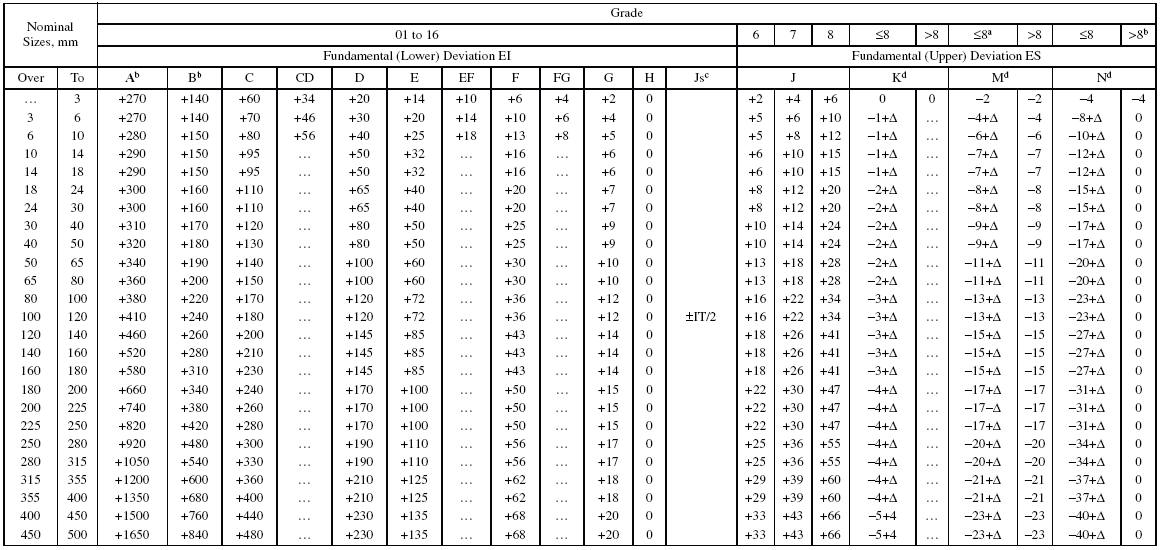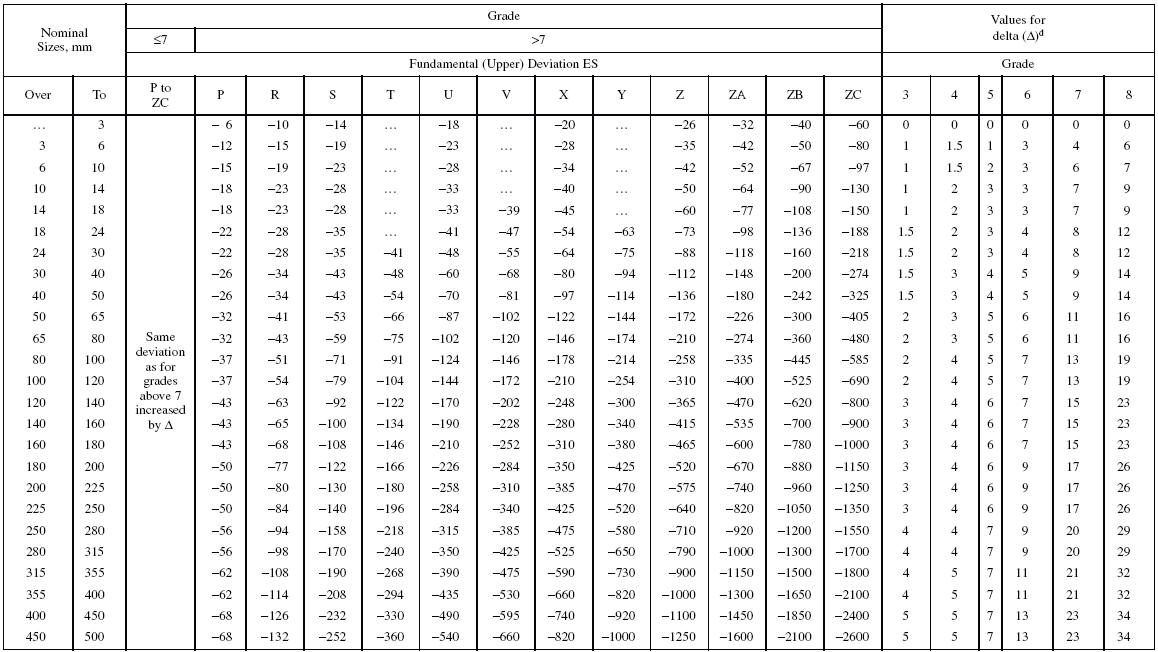British Standard Fundamental Deviations for Shafts
BS 4500:1969a Not applicable to sizes up to 1 mm.
ba c cd d e ef f fg g h js b
b In grades 7 to 11, the two symmetrical deviations ±IT/2 should be rounded if the IT value in micrometers is an odd value by replacing it with the even value immediately
below. For example, if IT = 175, replace it by 174.The dimensions are in 0.001 mm, except the nominal sizes, which are in millimeters.a Special case: for M6, ES = −9 for sizes from 250 to 315 mm, instead of −11.

b Not applicable to sizes up to 1 mm.

c In grades 7 to 11, the two symmetrical deviations ±IT/2 should be rounded if the IT value in micrometers is an odd value, by replacing it with the even value below. For example, if IT = 175, replace it by 174.

d When calculating deviations for holes K, M, and N with tolerance grades up to and including IT8, and holes P to ZC with tolerance grades up to and including IT7, the delta (Δ) values are added to the upper deviation ES. For example, for 25 P7, ES = −0.022 + 0.008 = −0.014 mm.The dimensions are given in 0.001 mm, except the nominal sizes, which are in millimeters.

Recherche personnalisée